# How are Beams constructed?

So recently I’ve been attempting to make my own development process easier via using Beams to construct roads. My first attempt was to simply get the Cubic Bezier from the Beam’s values and re-construct it from Parts equal to Beam’s Segments.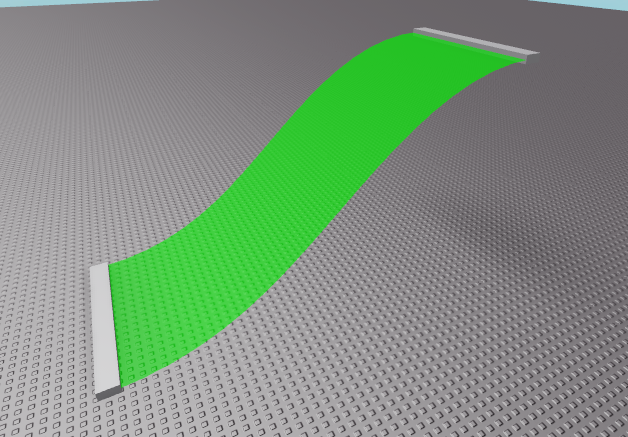The method above just places bricks to fill in the Beam. This method works perfectly fine for very simple pieces of road like this one. But if we add complexity by curving it.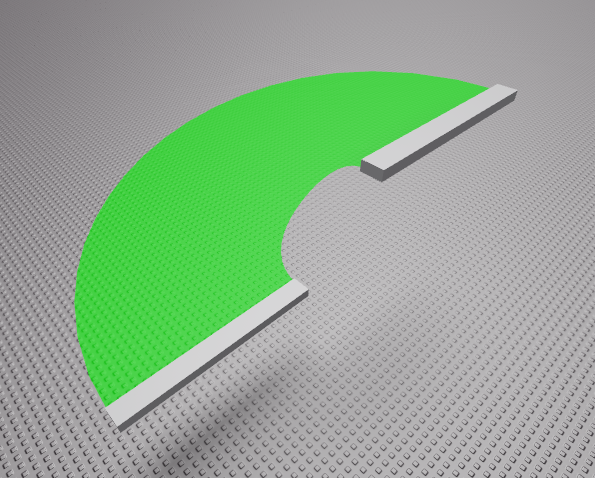We can see that using normal bricks isn’t the way to do it. So I attempted to use Polygons instead. The Polygon is roughly the following: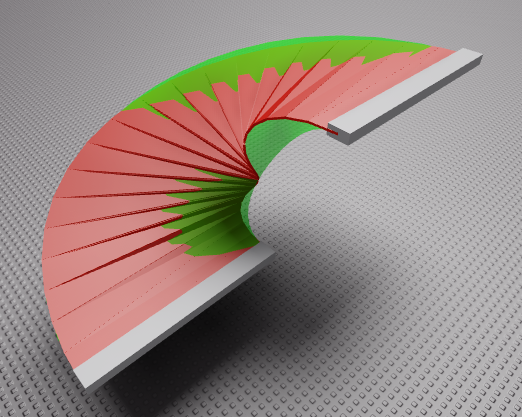Here we find a different issue. It appears the Path doesn’t have correct orientation. This could also be seen from the previous example where normal bricks were used.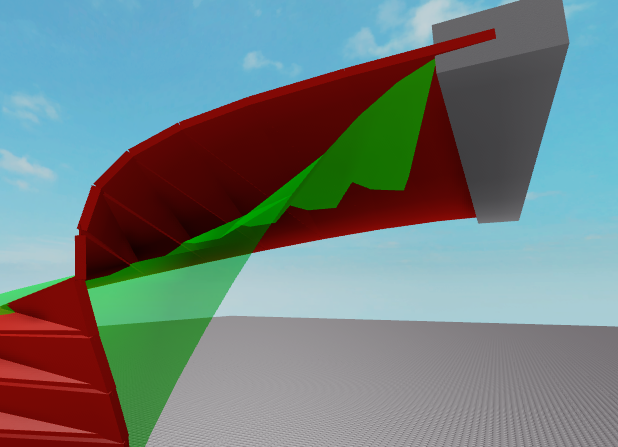I know for sure that I’m creating a Bezier Curve with correct values as seen from the following image: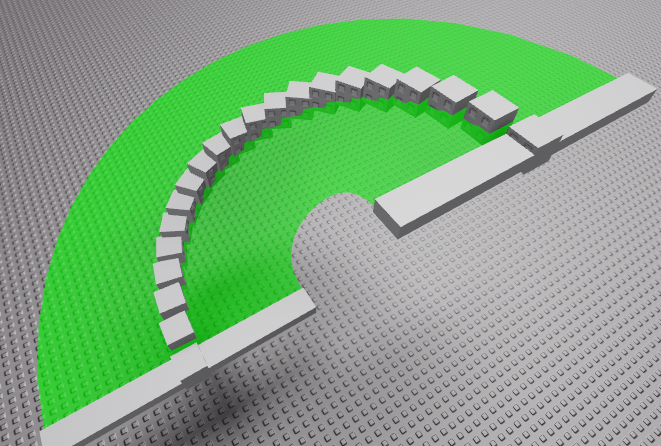All of those parts are perfectly aligned to the middle of the beam and the Start and End positions perfectly align with the start part and end part.

The Cubic Bezier function I’m using is the following:

``````function cubicBezier(t, p0, p1, p2, p3)
local l1 = p0:Lerp(p1, t)
local l2 = p1:Lerp(p2, t)
local l3 = p2:Lerp(p3, t)
local a = l1:Lerp(l2, t)
local b = l2:Lerp(l3, t)
return a:Lerp(b,t)
end
``````

parameter “t” being the step between 0 and 1 and the rest of the parameters go along with the following image taken from Roblox wiki.

Regardless of what I’ve tried. I just haven’t been able to nail it. I remember seeing a wiki article or a devforum post explaining how the beam is constructed but I can’t find that anymore either. Therefore I’d love to get more insight on what is the correct method for re-creating beams using Parts / Wedges.

Here is the place I’ve been using: Testing.rbxl (20.9 KB)

6 Likes

It looks like your part orientation is coming from interpolating the CFrames, which is a natural way to make a non-“banked” ramp or stairway, but it isn’t the same as the beam, which has torsion. To follow the beam, I think you’d want the parts’ CFrames to be the full Frenet-Serret Frame of the spline, also called the TNB Frame. This is the orthonormal basis you get from the unit Tangent (first derivative of the curve as a unit vector, aka the motion direction), the unit Normal (normalized second derivative of the curve, also the first vector derivative of the tangent) and their cross product, the unit Binormal. This coordinate frame should orient to the sloped surface of the beam (how closely depends on the number of segments in your beam, and what approximation methods and parameterization the beam code uses internally).

The derivatives you want are properly those of the curve in its arc-length parameterized form. The normalized tangent will be the same at some point for any parameterization, but to sample the curve uniformly and get the normals right, you need the arc length parameterization. For a cubic spline, arc length doesn’t have a closed-form solution you can just get as a polynomial and take derivatives of, since the exact arc length is the solution to an elliptic integral. But, you can easily get good approximations to the derivatives numerically, just by first and second differences for small, discrete steps along the arc-length-parameterized spline (the spline positions at distance s and s + ds for some small ds) The vector from p(s) to p(s+ds) is your tangent approximation, and the normal approximation is the difference between the tangents at point p(s) and p(s+ds). Cross them, and that’s your binormal. Because these are approximations, they will not all be perfectly orthogonal, but after you use CFrame.FromMatrix(p,N,B,-T) and set the part CFrame, Roblox’s internal orthonormalization of the CFrame will correct this.

Edit: I changed the variable in the paragraph above from t to s because I realized after I wrote it that there was potential to confuse this parameter with the t you’re using with Lerp. s is the parameter for the curve parameterized by arc length, which is not the same as your linear parameter t. You need to approximate s for a given t by numerical methods (searching). Stepping by tiny nudges of the linear t and accumulating the distance between the points gives you a cheap-ish arc length approximation you can uniformly resample with linear interpolation to get your mapping of values of t that correspond to uniform steps of s along the curve. @EgoMoose has a post devoted to this which includes more accurate (at the expense of more computation) options for this s(t) arc-length reparameterization : Reparameterization: Tips and Tricks

4 Likes

Thank you for the extremely in-depth response!This will take me a while to process but I’ll be sure try this out and put the results here!

Alright, apologies for the delay.

I haven’t quite been able to nail the process.
Using the TNB Frame the results I get are always rotated and doesn’t quite follow the beam.

The maths should be correct. But due to the curve the entire beam turns sideways.

Another finding I found was when using the TNB frame created

As seen from the image, using the TNB frame created makes the part be rendered inside out. This might be an engine bug, or the CFrame is somewhat invalid.

Here is an updated place file: Testing.rbxl (23.2 KB)
in the game.ServerScriptService.Script removing comments on lines 98 and 120 and commenting lines from 124 to 132 will show results of the first image.

Again, apologies for the long delay and thank you in advance

I will take a look as soon as I have a chance. It looks like this is going to need to be an additional constraint or offset so that the binormals match the Attachment orientations at the end. The Frenet frame alone is not going to just nicely be horizontal at the ends like your blocks, it does exactly what you’ve discovered, it matches the plane of the curve. But I’m going to have to think about what the twist amount to correct this properly needs to be.

1 Like

Yea I’ve gotten help from a few people and the closest we have gotten has been using 3D transformation and Quaternion slerp due to the ends not aligning properly with the start or end CFrames. The issue here was that it would work as long as the angle difference was lower than 180 degrees as the Quaternion Slerp we used would always turn as little as it can (meaning in roblox you can make a beam from -179deg to 179deg and it will travel the entire 358 degrees to the destination. The Slerp would only turn 2 degrees).

But yes, more investigation needed. Thank you for your support!

Yeah, I see your problem too. I tried using the beam orientation for the binormal, and like you say, it handles works for some cases, but not where the beam decides to twist more than 180 (my experimental changes attached). I will see if I can figure out what logic it’s using to determine the overall twist, because the beam flips back and forth a lot as I rotate either of the attachments.BeamProblem.rbxl (23.2 KB)

1 Like